Date: Tue, 11 Mar 1997 23:08:12 -0800 (PST)
From: Shuling
Subject: Mathematical Induction and the derivative

"Obtain a formula for the nth derivative of the product of two functions, and prove the formula by induction on n."

Any educated tries are appreciated.

Hi Shuling

If you take two functions, f and g, and find the first, second and third derivative of fg you should see the binomial coeficients and come up with a hypothesis that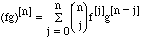.

Here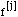designates the jth derivative of f and.

It is clear that this is valid if n=1 and hence for the induction step calculate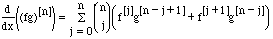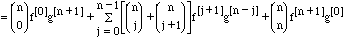But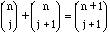so we get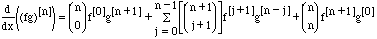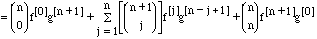Since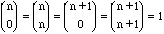we can write this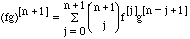which is the hypothezised expression for the (n+1)st derivative of fg. Thusfor all positive integers n.

Cheers
Penny

Go to Math Central

To return to the previous page use your browser's back button.